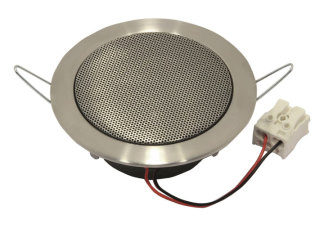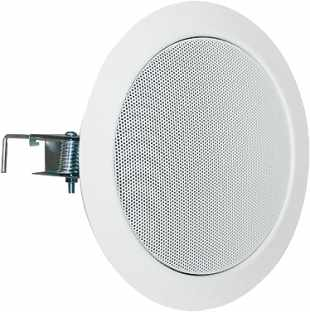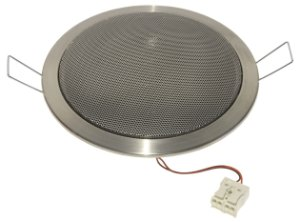# Visaton

With 40 years of experience in the field of acoustics and loudspeaker technology Visaton is a future oriented Company. With high engagement in research and development plus the high quality standards of production, Visaton is a specialised and world wide successfully operating German loudspeaker manufacturer.

## in Wall## Visaton DL 5 8 Ohm

order no. V-50097

EUR 23,42excl. VAT: € 19.68 / \$ 21.26

.

• power handling (continuous/programme) = 4/10 W
• frequency range = 150-20000 Hz
• resonance frequency fs = 370 Hz
• impedance R = 8 Ohm
• sound pressure level SPL = 84 dB (2,83V; 1m)
• DC resistance Re = 6,7 Ohm
• voice coil inductance L = 0,5 mH
• effective piston radiating area Sd = 12 cm2
• effective mechanical mass incl. air load mms = 0,5 g
• equivalent volume of compliance Vas = 0,19 l
• total Q factor Qts = 1,24 (Qms=2,48, Qes=2,5)
• voice coil diameter = 14 mm
• mounting diameter d = 53 mm
• overall diameter d = 85,5 mm
• mounting depth (not countersunk) t = 31 mm## Visaton DL 8 8 Ohm s

order no. V-50178

EUR 30,96excl. VAT: € 26.02 / \$ 28.10

.

• power handling (continuous/programme) = 10/30 W
• impedance R = 8 Ohm
• total Q factor Qts = (Qms=, Qes=1,43)## Visaton DL 8 8 Ohm w

order no. V-50098

EUR 30,96excl. VAT: € 26.02 / \$ 28.10

.

• power handling (continuous/programme) = 10/30 W
• impedance R = 8 Ohm
• total Q factor Qts = (Qms=, Qes=1,43)

## Visaton DL8 100V

order no. V-50198

EUR 44,19excl. VAT: € 37.13 / \$ 40.11

. The diaphragm is varnished with a hydrophabic coat.

• power handling (continuous/programme) = 3/1,5/0,75 W
• frequency range = 90-18000 Hz
• sound pressure level SPL = 87 dB (2,83V; 1m)
• voice coil diameter = 14 mm
• mounting diameter d = 93 mm
• overall diameter d = 105 mm
• mounting depth (not countersunk) t = 74 mm
• mass m = 0,515 kg## Visaton DL8ES

order no. V-50180

EUR 49,63excl. VAT: € 41.71 / \$ 45.04

.

• power handling (continuous/programme) = 10/30 W
• frequency range = 100-20000 Hz
• resonance frequency fs = 173 Hz
• impedance R = 8 Ohm
• sound pressure level SPL = 84 dB (2,83V; 1m)
• sound pressure level SPL = 84 dB (1W; 1m)
• DC resistance Re = 6,7 Ohm
• voice coil inductance L = 0,48 mH
• effective piston radiating area Sd = 31 cm2
• total Q factor Qts = 0,94 (Qms=2,72, Qes=1,43)
• voice coil diameter = 20 mm
• mounting diameter d = 60,5 mm
• overall diameter d = 105 mm
• mounting depth (not countersunk) t = 38 mm
• mass m = 0,33 kg
PDF data sheet## Visaton DL10ES

order no. V-50012

EUR 71,00excl. VAT: € 59.66 / \$ 64.44

.

• power handling (continuous/programme) = 20/30 W
• frequency range = 75-20000 Hz
• resonance frequency fs = 140 Hz
• impedance R = 8 Ohm
• sound pressure level SPL = 86 dB (2,83V; 1m)
• sound pressure level SPL = 86 dB (1W; 1m)
• voice coil diameter = 20 mm
• mounting diameter d = 60,5 mm
• overall diameter d = 135 mm
• mounting depth (not countersunk) t = 43 mm
• mass m = 0,4 kg

## Visaton DL10 100V

order no. V-50111

EUR 50,18excl. VAT: € 42.17 / \$ 45.54

. The diaphragm is varnished with a hydrophabic coat.

• power handling (continuous/programme) = 6/3/1,5 W
• frequency range = 75-20000 Hz
• resonance frequency fs = 140 Hz
• sound pressure level SPL = 86 dB (2,83V; 1m)
• voice coil diameter = 20 mm
• maximum peak linear excursion vibration xlin = +/- 2,5 mm
• mounting diameter d = 110 mm
• overall diameter d = 135 mm
• mounting depth (not countersunk) t = 76 mm
• mass m = 0,545 kg

## Visaton DL10 8 Ohm w

order no. V-50110

EUR 37,47excl. VAT: € 31.49 / \$ 34.01

. The diaphragm is varnished with a hydrophabic coat.

• power handling (continuous/programme) = 20/30 W
• frequency range = 75-20000 Hz
• resonance frequency fs = 140 Hz
• impedance R = 8 Ohm
• sound pressure level SPL = 86 dB (2,83V; 1m)
• voice coil diameter = 20 mm
• maximum peak linear excursion vibration xlin = +/- 2,5 mm
• mounting diameter d = 110 mm
• overall diameter d = 135 mm
• mounting depth (not countersunk) t = 43 mm
• mass m = 0,4 kg

## Visaton DL10 8 Ohm s

order no. V-50014

EUR 37,47excl. VAT: € 31.49 / \$ 34.01

. The diaphragm is varnished with a hydrophabic coat.

• power handling (continuous/programme) = 20/30 W
• frequency range = 75-20000 Hz
• resonance frequency fs = 140 Hz
• impedance R = 8 Ohm
• sound pressure level SPL = 86 dB (2,83V; 1m)
• voice coil diameter = 20 mm
• maximum peak linear excursion vibration xlin = +/- 2,5 mm
• mounting diameter d = 110 mm
• overall diameter d = 135 mm
• mounting depth (not countersunk) t = 43 mm
• mass m = 0,4 kg## Visaton DL13/2T 8 Ohm

order no. V-50162

EUR 56,69excl. VAT: € 47.64 / \$ 51.45

16 cm coax driver with high quality cone.

• power handling (continuous/programme) = 30/50 W
• frequency range = 100-20000 Hz
• resonance frequency fs = 150 Hz
• impedance R = 8 Ohm
• sound pressure level SPL = 98 dB (2,83V; 1m)
• voice coil diameter = 25 mm
• mounting diameter d = 165 mm
• overall diameter d = 180 mm
• mounting depth (not countersunk) t = 8,8 mm
• mass m = 0,86 kg## Visaton DL13/2ES

order no. V-50163

EUR 105,90excl. VAT: € 88.99 / \$ 96.11

.

• power handling (continuous/programme) = 30/50 W
• frequency range = 100-20000 Hz
• resonance frequency fs = 150 Hz
• impedance R = 8 Ohm
• sound pressure level SPL = 89 dB (2,83V; 1m)
• sound pressure level SPL = 89 dB (1W; 1m)
• mounting diameter d = 70 mm
• overall diameter d = 165 mm
• mounting depth (not countersunk) t = 51,5x2,7 mm
• mass m = 0,61 kg

## Visaton DL13/2T 8Ohm

order no. V-50162

EUR 56,69excl. VAT: € 47.64 / \$ 51.45

17 cm coax driver with high quality cone.

• power handling (continuous/programme) = 30/50 W
• frequency range = 100-20000 Hz
• resonance frequency fs = 150 Hz
• impedance R = 8 Ohm
• sound pressure level SPL = 89 dB (2,83V; 1m)
• voice coil diameter = 25 mm
• mounting diameter d = 165 mm
• overall diameter d = 180 mm
• mounting depth (not countersunk) t = 88 mm
• mass m = 0,86 kg

## Visaton DL18/2T 8 Ohm

order no. V-50100

EUR 67,48excl. VAT: € 56.71 / \$ 61.24

21 cm coax driver with high quality cone. The diaphragm is varnished with a hydrophabic coat.

• power handling (continuous/programme) = 50/70 W
• frequency range = 50-20000 Hz
• impedance R = 8 Ohm
• sound pressure level SPL = 90 dB (2,83V; 1m)
• DC resistance Re = 6,9 Ohm
• voice coil inductance L = 0,75 mH
• effective piston radiating area Sd = 129 cm2
• effective mechanical mass incl. air load mms = 7,9 g
• equivalent volume of compliance Vas = 15 l
• total Q factor Qts = 1,03 (Qms=2,68, Qes=1,68)
• voice coil diameter = 25 mm
• mounting diameter d = 200 mm
• overall diameter d = 218 mm
• mounting depth (not countersunk) t = 93 mm
• mass m = 1,15 kg

## Visaton DL18/2 100 V

order no. V-50104

EUR 85,39excl. VAT: € 71.76 / \$ 77.50

21 cm coax driver with high quality cone. The diaphragm is varnished with a hydrophabic coat.

• power handling (continuous/programme) = 20/10/5 W
• frequency range = 50-20000 Hz
• resonance frequency fs = 90 Hz
• sound pressure level SPL = 90 dB (2,83V; 1m)
• voice coil diameter = 25 mm
• mounting diameter d = 200 mm
• overall diameter d = 218 mm
• mounting depth (not countersunk) t = 93 mm
• mass m = 1,5 kg

## Visaton DL18/2SQ 8 Ohm/100V

order no. V-50154

EUR 55,76excl. VAT: € 46.86 / \$ 50.61

.

• power handling (continuous/programme) = 40/60; 6/3/1,5 W
• resonance frequency fs = 100 Hz
• impedance R = 8 Ohm
• sound pressure level SPL = 90 dB (2,83V; 1m)
• voice coil diameter = 20 mm
• mounting diameter d = 187x187 mm
• overall diameter d = 220x220 mm
• mounting depth (not countersunk) t = 63 mm
• mass m = 0,864 kg

## Visaton DL18/1 100V RAL9010

order no. V-50105

EUR 46,78excl. VAT: € 39.31 / \$ 42.46

. The diaphragm is varnished with a hydrophabic coat.

• power handling (continuous/programme) = 6/3/1,5 W
• frequency range = 70-20000 Hz
• resonance frequency fs = 100 Hz
• sound pressure level SPL = 93 dB (2,83V; 1m)
• voice coil diameter = 20 mm
• mounting diameter d = 200 mm
• overall diameter d = 218 mm
• mounting depth (not countersunk) t = 93 mm
• mass m = 1,15 kg

## Visaton DL18/1 100V RAL9016

order no. V-50106

EUR 46,78excl. VAT: € 39.31 / \$ 42.46

. The diaphragm is varnished with a hydrophabic coat.

• power handling (continuous/programme) = 6/3/1,5 W
• frequency range = 70-20000 Hz
• resonance frequency fs = 100 Hz
• sound pressure level SPL = 93 dB (2,83V; 1m)
• voice coil diameter = 20 mm
• mounting diameter d = 200 mm
• overall diameter d = 218 mm
• mounting depth (not countersunk) t = 93 mm
• mass m = 1,15 kg

## Wall## Visaton WL13N 100V

order no. V-50302

EUR 57,27excl. VAT: € 48.13 / \$ 51.98

28x17 cm full range driver with high quality cone.

• power handling (continuous/programme) = 6/3/1,5 W
• frequency range = 90-20000 Hz
• sound pressure level SPL = 88 dB (2,83V; 1m)
• overall diameter d = 170x280 mm
• mounting depth (not countersunk) t = 65 mm
• mass m = 1,4 kg

## Visaton WL13NR 100V

order no. V-50303

EUR 63,93excl. VAT: € 53.72 / \$ 58.02

28x17 cm full range driver with high quality cone.

• power handling (continuous/programme) = 6/3/1,5 W
• frequency range = 90-20000 Hz
• sound pressure level SPL = 88 dB (2,83V; 1m)
• overall diameter d = 170x280 mm
• mounting depth (not countersunk) t = 65 mm
• mass m = 1,4 kg

## Visaton WL13P 100V

order no. V-50321

EUR 59,23excl. VAT: € 49.77 / \$ 53.75

28x17 cm full range driver with high quality cone.

• power handling (continuous/programme) = 6/3/1,5 W
• frequency range = 90-16000 Hz
• sound pressure level SPL = 88 dB (2,83V; 1m)
• overall diameter d = 170x280 mm
• mounting depth (not countersunk) t = 61 mm
• mass m = 1,15 kg

## Visaton WL13PR 100V

order no. V-50323

EUR 70,14excl. VAT: € 58.94 / \$ 63.66

28x17 cm full range driver with high quality cone.

• power handling (continuous/programme) = 6/3/1,5 W
• frequency range = 90-16000 Hz
• sound pressure level SPL = 88 dB (2,83V; 1m)
• overall diameter d = `170x280 mm
• mounting depth (not countersunk) t = 61 mm
• mass m = 1,15 kg

The cabinet volume calculations are executed considering an external resistance of 0.3 Ohm. We are using, if no other data is known or available, the information supplied by the manufacturer.

Please note: the frequency response doesn't say much about the cut-off frequency inside the cabinet! Therefore, we mostly give you 2 examples with dimensioning considerations and an indication, how low the loudspeaker will reproduce.

Home